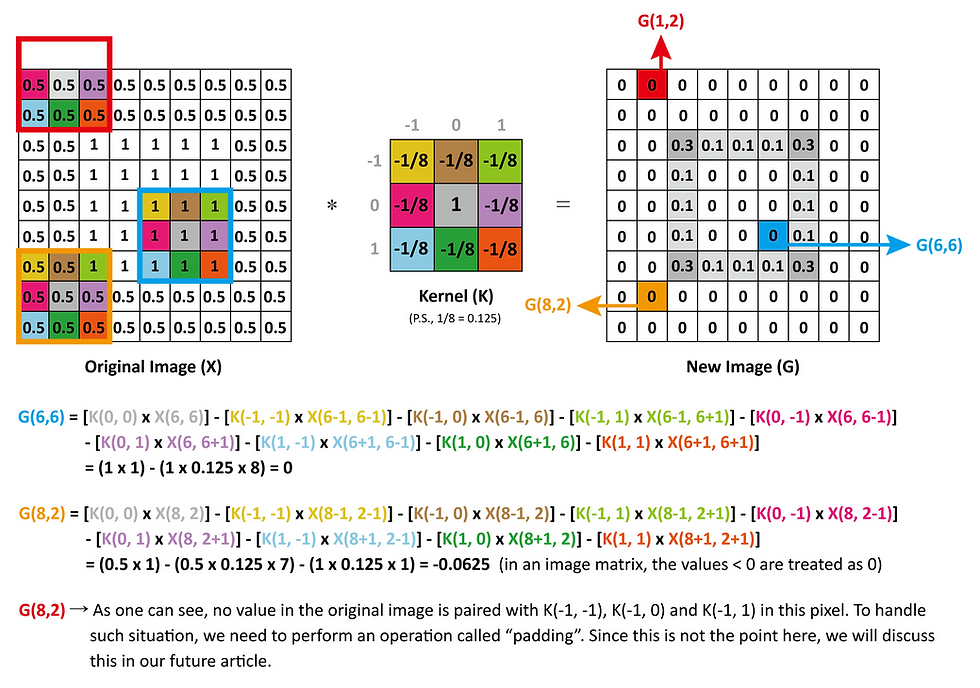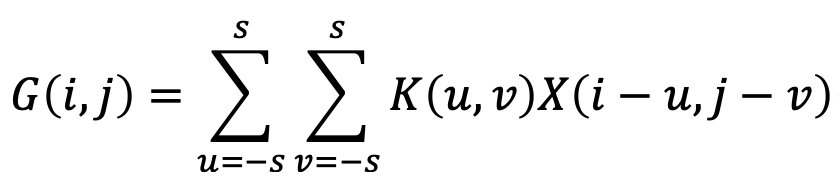top of pageSearch

# What is convolution? A brief explanation.

Updated: Aug 27, 2019Since convolutional neural network is getting popular, the term “convolution” also becomes familiar to many people. But just what exactly is convolution? This article will answer this question for those who are willing to expand their knowledge in the mathematical field.

## The definition of convolution

If you have two functions, f(x) and g(x), and you’d like to generate a third function based on them, there are actually multiple measures you can choose from. For instance, function composition is an option to go with, which can produce a new function equals f(g(x)). Similarly, “convolution” is one of such mathematical operations allowing one to generate a new function out of two existed functions. The mathematical operation is defined as follows:

• For continuous situation:• For discrete situation (discrete convolution):We now know the definition of “convolution”, but what does it really means in the real world? The answer to this question will be discussed in the next paragraph.

## The physical meaning of math

Before we go further with convolution, we’d like to propose a concept: in many cases, a mathematical equation does not have an ultimate physical meaning. Takes “1+1 = 2” as an example, the equation can mean many things. For instance, one orange plus one orange equals two oranges, or one person plus one person equals two people. Note that these are the situations where “1+1 = 2” is applicable, but there are also some situations where “1+1 = 2” does not hold true. For example, one drop of water plus another drop does not necessarily equal two drops of water (they may merge into a larger drop). We can also say that any condition that matches the depiction of “1+1 = 2” is a potential physical meaning for the equation, so our interpretation of this equation changes accordingly depending on which condition we’re currently referring to.

Similarly, “convolution” can be understood in many fashions, depending on the area it’s applied to. In the rest of this article, we’re going to introduce two important applications of convolution in signal and image processing, respectively. And as you are about to see, they are very distinct in respect of their physical meanings.

## Example of application 01 – Signal Processing

Given that you have a linear, time-invariant (LTI) system capable of turning an input signal, which can be described by the function x(t), into the output signal, which can be described by y(t) (P.S., y(t) is also known as the response of x(t)), you’ll find out that the response, y(t), is equal to the convolution between the input signal and a special kind of response called the impulse response. In other words:where the function h represents impulse response.Figure 1. For the equation above to be valid, the system must be linear and time-invariant (LTI).

To understand why the equation above is true, some prior knowledge is necessary, including (1) what is an impulse and impulse response, (2) what is sifting property and (3) what is a linear time-invariant system. These topics are discussed in below:

(1) What is an impulse and impulse response?

If you have a linear time-invariant system capable of processing signal (i.e., taking in an input signal and generate a response signal correspondingly), how can you have a full understanding about the system?

Ideally, we can input signals with all possible frequencies to the system at the same time and see what kind of response it produces. And since we have observed how the system reacts to all frequencies, we’ve already fully understood how the system behaves.

An impulse represented by the function δ (Dirac delta function, which will be introduced later) is just the kind of signal that matches the description above, which allows us to fully understand a LTI system. As we can see from Figure 2 (a), δ’s value remains to be 1 on the entire frequency domain, meaning that it contains inputs of all frequencies. And since these frequencies are all applied to the system at the same time, it’s natural to expect that the function δ on the time domain has value solely on a certain time point (in this case, t = 0), and its value at that time point reaches to infinity (as shown in Figure 2 (b). Also notice that after we apply an impulse to a LTI system, the system will produce a corresponding response; such response is called an impulse response (as shown in Figure 2 (c)).Figure 2. Impulse, described by Dirac delta function, and impulse response. (a) An impulse drawn on the frequency domain. (b) An impulse drawn on the time domain. (c) The relationship between an impulse and impulse response (P.S., the system is linear and time-invariant).

As mentioned previously, an impulse can be described by a special function called Dirac delta function (denoted by “δ”), whose definition is as follows:The value of Dirac delta function at point other than t = 0 equals zero, and its value reaches to infinity at t = 0.

Such function has many intriguing properties. Two of them that are particularly important for the future discussion are the sifting property (it’ll be introduced in the next paragraph) and the fact that the “area” under the graph of δ(t) at the time point t = 0 is equal to 1. And since the geometrical meaning of integral is the area under the curve of a function, one can anticipate that (see Figure 3):where “n1” and “n2” can be any number, as long as the range of the definite integral includes 0.Figure 3. Explaining the definite integral of Dirac delta function.

(2) What is sifting property (note that it’s “sifting”, not “shifting”)?

In simple words, given that x(t) is any function and δ stands for Dirac delta function, sifting property states that:The reason for this equation to be valid is explained in Figure 4:Figure 4. Explaining sifting property.

(3) What is a linear and time-invariant system?

We must emphasize again that the conclusion, “the response, y(t), is equal to the convolution between its input signal, x(τ), and impulse response, h(t τ)”, only works when the system is a linear time-invariant (LTI) system, which is a system owning two important features: linear and time-invariant.

Figure 5 summarizes the properties of a LTI system:Figure 5. The properties of a linear time-invariant (LTI) system (P.S., k is any number; x(t) is the input signal while y(t) is its output). (a) if the input of a linear system is the linear combination of several inputs, the output will be the linear combination of the responses of these inputs. (b) Shifting in time by value k in the input will cause the response to shift in time by the same value.

With the knowledge said, we can finally get to the point: understanding why an output signal is equal to the convolution between its input and impulse response. The following diagram demonstrates the deduction process for gaining such conclusion:Figure 6. The deduction process for gaining the conclusion: “to a LTI system, the output signal equals the convolution between its input and impulse response.”

• From (a) to (b): Since the system is time-invariant, we can shift both side in time by the value τ.

• From (b) to (c): Since the system is linear, we can multiply both side with a value x(τ) (i.e., the value of the function x(t) when t = τ; τ can be any number).

• From (c) to (d): Since the system is linear, we can integrate both side with respect to τ (note that the concept of integration is closely related to summation).

• From (d) to (e): According to the sifting property, the left side of the system is equal to x(t). And since by definition applying x(t) to the LTI system will give rise to the response y(t), we can now conclude that y(t) is equal to the convolution between x(τ) and h(t τ).

## Example of application 02 – Image Processing

For people who are studying machine learning, this application of convolution is critical since it is used in convolutional neural networks (CNNs). But how is it connected to the definition of the discrete convolution described on the top? Before we get to that, let’s first explore how convolution “looks like” in CNN and image processing.

In a picture containing meaningful objects, pixels are usually correlated with each other (especially those in the vicinity) in some fashions instead of randomly distributed on the image. To catch such correlation between a pixel and its neighbors, we can load an image and carry out some mathematical operation to each pixel on that image, combining its value with the values from the nearby pixels in some meaningful way, and as a result giving rise to a new image that is capable of illustrating the pixel correlation we’re looking for. Note that in order to do so, we’ll need another smaller, typically square, matrix called a kernel. Figure 7 demonstrates an example of how to apply a 3×3 kernel to a 9×9 black-and-white image to give rise to a new 9×9 black-and-white image for showing a certain feature of the original:Figure 7. We use 3 example pixels to demonstrate how to calculate the value of each pixel in the new image (G) from the original image (X) with the help of a kernel (K). In this case, the original image is a white square on a gray background. And as we can see, after being processed, only the pixels on the edges of the square have values; other pixels’ intensities are all equal to zero. Such processing is thus called “edge detection”.

As shown in Figure 7, the kernel with the central value equals 1 and other -1/8 is capable of showing the “edge” of the objects within the original image (i.e., performing edge detection). As a matter of fact, by altering the size of a kernel and the values in it, one can get a new image showing different information about the original picture, or even adding a certain visual effect to it (such as sharpening the image or making it looks embossing).

So, how exactly is the said process connects to convolution? Let’s try to describe the process with a generalized mathematical expression, and you’ll see. Given that we have a n × n image (denoted by “X”) and a k × k kernel (denoted by “K”), the value of a certain pixel on the n × n new image (denoted by “G”, which is generated by applying the kernel to the original matrix) can be depicted by the following equation, where s equals the quotient of k divided by 2 (see Figure 7):It’s clear now that the equation shown is a two-dimensional version of the discrete convolution aforementioned. In other words, we can say that “the value of the pixels on the new image equals the convolution between the original image and a kernel”, and that’s exactly why the neural network that adopts such process is called a “convolutional” neural network.

FYI, There is a similar process called cross-correlation that yields a result completely identical with convolution in the application of image processing (P.S., note that this is not true in the case of signal processing). The mathematical expression of cross-correlation is as follows: